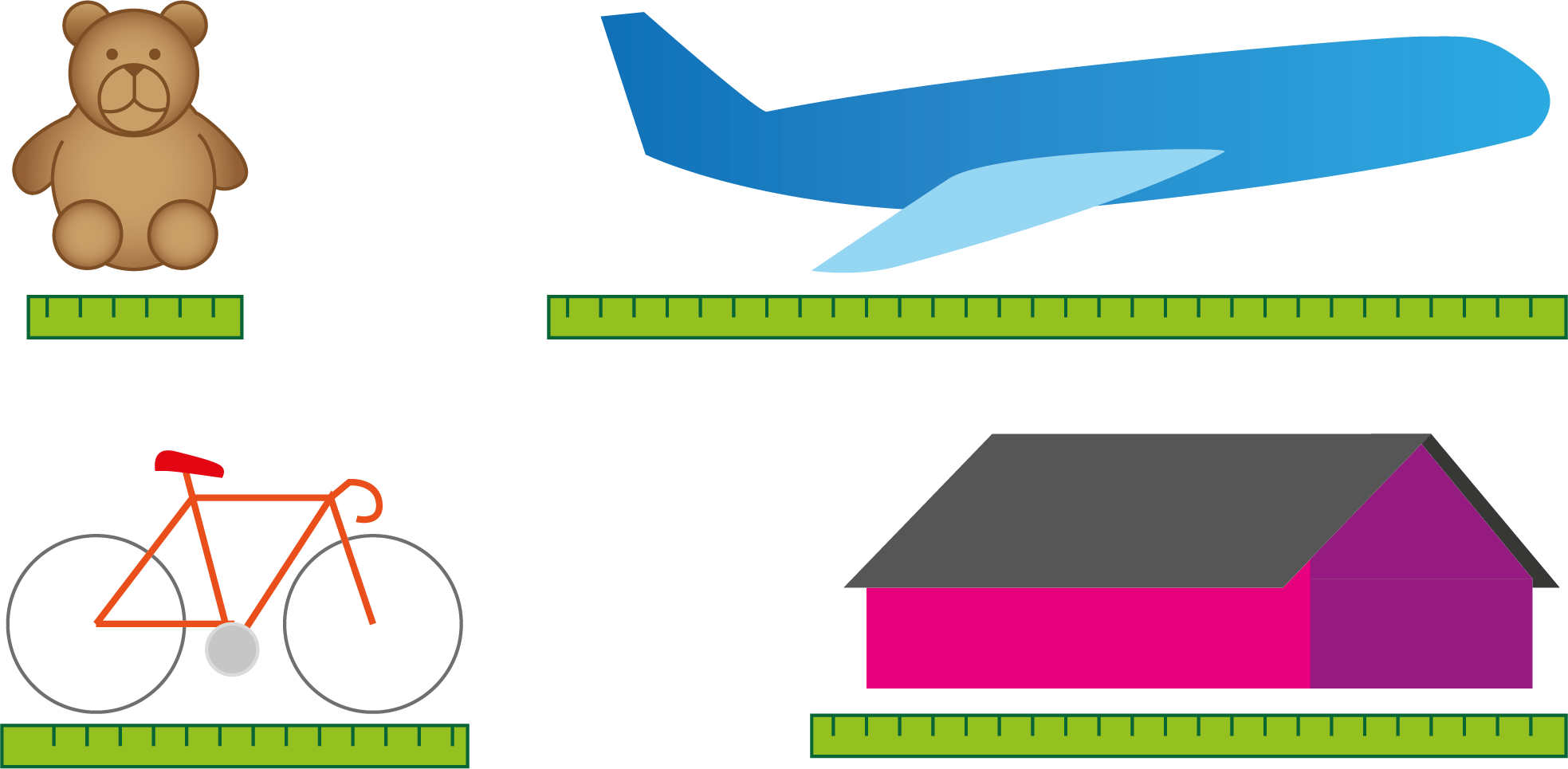# What Are the Metric Units for Length?

This entry uses the metric system. For the imperial system click here.

When you want to describe the size of a line, you’re talking about length. An airplane is longer than a teddy bear, and a house is longer than a bicycle. You can try to imagine how many bicycles you need for them to be as long as a house if you add them all together. You can also try to imagine how many teddy bears you need to make a line of teddy bears that is as long as an airplane.

However, what if your teddy bear is really big, but your friend’s teddy bear is really small? Then your measurement and their measurement can be very different from each other. To solve these kinds of problems, scientists agreed on a length we call a meter. When everyone uses meters when they measure the length of something, we can compare lengths to each other. As long as you know how long a meter is, you can measure the length of an airplane, a teddy bear, a house or a bicycle and see which one is the longest.

In school, you’ll use a ruler to measure length.Length is measured in meters, but we often want to measure something that is much shorter than a meter. For that reason, the meter is divided into smaller measurements called decimeters, centimeters and millimeters.

Deci- means tenth. When you divide a meter into $10$ equal pieces, the length of each piece is one decimeter (dm).

Centi- means hundredth. When you divide a meter into $100$ equal pieces, the length of each piece is one centimeter (cm).

Milli- means thousandth. When you divide a meter into $1000$ equal pieces, the length of each piece is one millimeter (mm).If you want to measure something that is much longer than a meter, you measure it in kilometers (km). Kilo- means thousand, which means that one kilometer is $1000$ meters.## Doing Calculations With Units of Length

Another word for a measure is a unit, and this is the most commonly used term. The bubble figure below will be very helpful when you understand how it works. The figure shows you what to multiply and divide by to move from one unit to another. For example, if you want to go from meters to centimeters, you have to multiply by $10$ twice. That’s the same as multiplying by $100$, because $10\cdot 10=100$.When you need to switch from one unit to another, you can let the bubble figure help you with the calculation. Remember that there are $100$ centimeters in $1$ meter, so you have to multiply/divide by $100$ when you switch between meters and centimeters. When learning to switch between units, it might be useful to you to read about powers of 10.

Example 1

Meters $⇔$ kilometers: $\begin{array}{llll}\hfill 5000\phantom{\rule{0.17em}{0ex}}\text{m}& =\left(5000÷1000\right)\phantom{\rule{0.33em}{0ex}}\text{km}\phantom{\rule{2em}{0ex}}& \hfill & \phantom{\rule{2em}{0ex}}\\ \hfill & =5\phantom{\rule{0.17em}{0ex}}\text{km}\phantom{\rule{2em}{0ex}}& \hfill & \phantom{\rule{2em}{0ex}}\\ \hfill 3\phantom{\rule{0.17em}{0ex}}\text{km}& =\left(3\cdot 1000\right)\phantom{\rule{0.33em}{0ex}}\text{m}\phantom{\rule{2em}{0ex}}& \hfill & \phantom{\rule{2em}{0ex}}\\ \hfill & =3000\phantom{\rule{0.17em}{0ex}}\text{m}\phantom{\rule{2em}{0ex}}& \hfill & \phantom{\rule{2em}{0ex}}\end{array}$

Meters $⇔$ centimeters: $\begin{array}{llll}\hfill 2\phantom{\rule{0.17em}{0ex}}\text{m}& =\left(2\cdot 100\right)\phantom{\rule{0.33em}{0ex}}\text{cm}\phantom{\rule{2em}{0ex}}& \hfill & \phantom{\rule{2em}{0ex}}\\ \hfill & =200\phantom{\rule{0.17em}{0ex}}\text{cm}\phantom{\rule{2em}{0ex}}& \hfill & \phantom{\rule{2em}{0ex}}\\ \hfill 400\phantom{\rule{0.17em}{0ex}}\text{cm}& =\left(400÷100\right)\phantom{\rule{0.33em}{0ex}}\text{m}\phantom{\rule{2em}{0ex}}& \hfill & \phantom{\rule{2em}{0ex}}\\ \hfill & =4\phantom{\rule{0.17em}{0ex}}\text{m}\phantom{\rule{2em}{0ex}}& \hfill & \phantom{\rule{2em}{0ex}}\end{array}$

Decimeters $⇔$ meters: $\begin{array}{llll}\hfill 20\phantom{\rule{0.17em}{0ex}}\text{dm}& =\left(20÷10\right)\phantom{\rule{0.33em}{0ex}}\text{m}\phantom{\rule{2em}{0ex}}& \hfill & \phantom{\rule{2em}{0ex}}\\ \hfill & =2\phantom{\rule{0.17em}{0ex}}\text{m}\phantom{\rule{2em}{0ex}}& \hfill & \phantom{\rule{2em}{0ex}}\\ \hfill 34\phantom{\rule{0.17em}{0ex}}\text{m}& =\left(34\cdot 10\right)\phantom{\rule{0.33em}{0ex}}\text{dm}\phantom{\rule{2em}{0ex}}& \hfill & \phantom{\rule{2em}{0ex}}\\ \hfill & =340\phantom{\rule{0.17em}{0ex}}\text{dm}\phantom{\rule{2em}{0ex}}& \hfill & \phantom{\rule{2em}{0ex}}\end{array}$

Decimeters $⇔$ centimeters: $\begin{array}{llll}\hfill 8\phantom{\rule{0.17em}{0ex}}\text{dm}& =\left(8\cdot 10\right)\phantom{\rule{0.33em}{0ex}}\text{cm}\phantom{\rule{2em}{0ex}}& \hfill & \phantom{\rule{2em}{0ex}}\\ \hfill & =80\phantom{\rule{0.17em}{0ex}}\text{cm}\phantom{\rule{2em}{0ex}}& \hfill & \phantom{\rule{2em}{0ex}}\\ \hfill 90\phantom{\rule{0.17em}{0ex}}\text{cm}& =\left(90÷10\right)\phantom{\rule{0.33em}{0ex}}\text{dm}\phantom{\rule{2em}{0ex}}& \hfill & \phantom{\rule{2em}{0ex}}\\ \hfill & =9\phantom{\rule{0.17em}{0ex}}\text{dm}\phantom{\rule{2em}{0ex}}& \hfill & \phantom{\rule{2em}{0ex}}\end{array}$

Millimeters $⇔$ centimeters: $\begin{array}{llll}\hfill 700\phantom{\rule{0.17em}{0ex}}\text{mm}& =\left(700÷10\right)\phantom{\rule{0.33em}{0ex}}\text{cm}\phantom{\rule{2em}{0ex}}& \hfill & \phantom{\rule{2em}{0ex}}\\ \hfill & =70\phantom{\rule{0.17em}{0ex}}\text{cm}\phantom{\rule{2em}{0ex}}& \hfill & \phantom{\rule{2em}{0ex}}\\ \hfill 32\phantom{\rule{0.17em}{0ex}}\text{cm}& =\left(32\cdot 10\right)\phantom{\rule{0.33em}{0ex}}\text{mm}\phantom{\rule{2em}{0ex}}& \hfill & \phantom{\rule{2em}{0ex}}\\ \hfill & =320\phantom{\rule{0.17em}{0ex}}\text{mm}\phantom{\rule{2em}{0ex}}& \hfill & \phantom{\rule{2em}{0ex}}\end{array}$

Math Vault

Would you like to solve exercises about length? Try Math Vault!